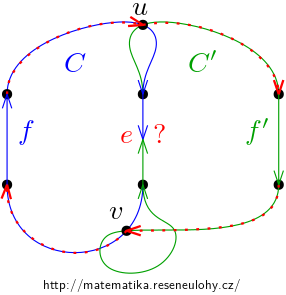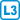## Total cyclic orientations

The orientation of the graph is totally cyclic if each edge lies on an directed circle. Prove that any graph $$G$$ has $$T_G (0{,}2)$$ totally cyclic orientations.

• #### Hint

Use the recurrent relation to calculate the Tutte polynomial.

• #### Solution

Let $$O (G)$$ be the number of totally cyclic orientations of the graph $$G$$. Substituting 0 and 2 into the Tutte polynomial we get the following relation:

$O (G) = \begin{cases} 0 & \textrm {if  e  is a bridge,} \\ 2O (G-e) & \textrm {if  e  is a loop,} \\ O (G-e) + O (G / e) & \textrm {if  e  is neither a bridge nor a loop.} \end{cases}$

For an empty graph then $$O (G) = 1$$.

The first two options are simple. A graph with a bridge cannot contain a totally cyclic one orientation due to the bridge. On the contrary, we can orient the loop in the graph in either of two possible ways and thus the number of orientations is twice larger than after removing a loop. The loop also has no effect on any existent orientation.

For the last relationship, it is necessary to analyze several cases. Let’s consider a totally cyclical orientation of the graph $$G$$ that the switching of the edge $$e$$ it will no longer be totally cyclic. Then it is a good orientation for $$G / e$$, but it does not count in $$G / e$$. If the edge direction can be switched, it is counted once in $$G / e$$ and once in $$G-e$$. Here it is necessary to test that if the edge is switchable, then after its removal, the orientation is still totally cyclic.

So we know that all the orientations of $$G$$ are counted. We still need to verify that we do not count anything extra.

If the totally cyclic orientation is common for $$G / e$$ and $$G-e$$, then to the orientation of the edge $$e$$ it does not matter and can be chosen in both ways.

If the orientation $$G / e$$ is totally cyclic but for $$G-e$$ is not, then there is an edge $$f$$, which in its cycle $$C$$ in $$G$$ forces the direction of the edge $$e$$ in a certain way.

If this orientation was not appropriate, so there is still an edge $$f ’$$, which in its cycle $$C’$$ forces the opposite orientation to the edge $$e$$, see the picture.But then from the common vertices of both cycles we choose the vertices $$u$$ and $$v$$, for example so that they are closest to the edge $$e$$ along the cycle $$C$$. However, $$u-f'-v-f-u$$ in the given orientation matches the orientations of the edges $$f$$ and $$f '$$ without passing through the edge $$e$$.

This cannot happen, so such an edge $$f ’$$ does not exist and the orientation or $$e$$ is determined unambiguously and the respective totally cyclic orientation is counted only once.

Orientations that would be totally cyclic in $$G-e$$, but not in in $$G / e$$ cannot exist, because by merging two vertices into one we do not interrupt any cycle; we at most split one into two.